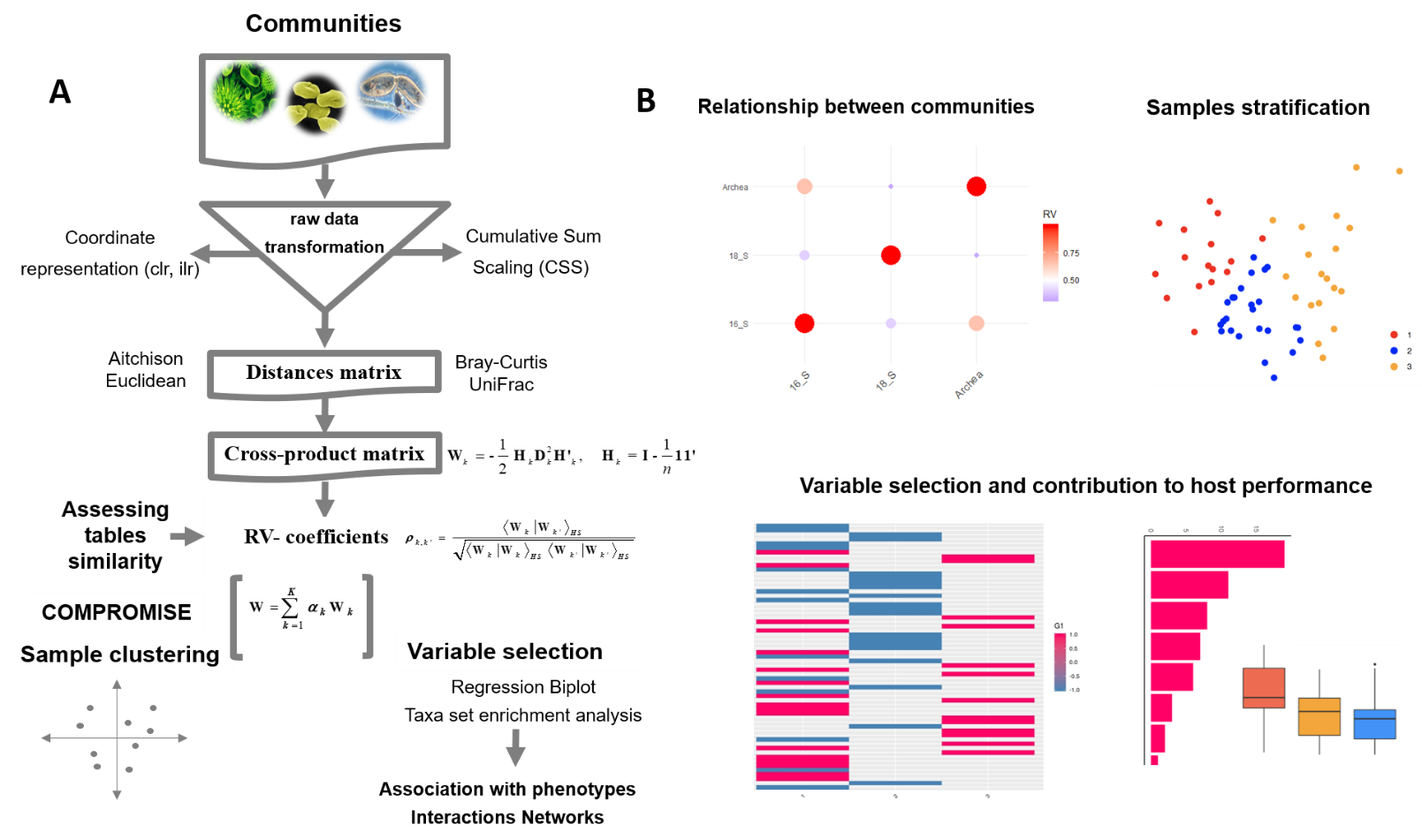# 1 Version Info

## 1.1 Introduction

Recent advances in omics technologies have revolutionized biological research providing vast amounts of heterogeneous data. The drop in ‘omics’ technologies costs enables collection of data from multiple sources; facilitating the study of gene expression, proteins, metagenomics and metabolites, and to evaluate their relationship with health and diseases status. However, the integration of these heterogeneous datasets is not a trivial task. Several statistical methods have been developed to handle these challenges (Mariette and Villa-Vialaneix 2017,@mixomics,@mofa). STATIS-ACT (Plantes 1976, @escoufier1976) is one of the methods capable of analyzing data arising from several configurations and to compare subspaces.

STATIS is a PCA extension to analyze multiple datasets of variables collected from the same individual or the same variables collected from multiple individuals (Lavit et al. 1994). The method was designed to integrate several datasets into an optimum space called compromise. However, STATIS has some constraint since can only be used with continuous data. In addition, the subspace generated by STATIS only can establish relationships between common elements in all datasets, i. e. observations (samples) or variables (genes/transcripts/proteins/metabolites/microbial communities), leading to additional drawbacks. Neither is it possible to establish relationships between observations and variables, nor can it provide a variable selection strategy.

Here, we present Link-HD, an R package to integrate multiple heterogeneous datasets based on STATIS. Our approach is a generalization of multidimensional scaling, which uses distance matrices for numerical, categorical or compositional variables instead of the raw data. Furthermore, the methodology was enhanced with Regression Biplot (Gabriel 1971,@gowerH) and differential abundance testing to perform variable selection and to identify OTUs that differ between two or more categories.

##Methods

Our package was designed to integrate multiple communities from a holistic view. It can deal with compositional data, and it includes centered log ratio and cumulative-sum scaling (CSS) (Paulson et al. 2013) transformations. Link-HD also incorporates variable selection and visualization tools which allows for a better undestunding of communities structure, and, their relationship with target traits.Link-HD pipeline overview. First, raw data are transformed using cumulative sum scaling (CSS) (Paulson et al. 2013) or centered log ratio transformation (clr) (Aitchison 1982). Second, distance and cross -product matrices are obtained to assess the similarity between datasets. Third, the compromise uses the first eigenvectors from RV to build the common configuration W matrix. The intra-structure step is the eigen-decomposition of W, where each observation is projected onto the common configuration. In this step, clusters of observations can be obtained. Finally, relationships between clusters and targets can identify selected variables responsible for the attained structure. (B) Link-HD includes visualization tools to explore relationships between data. In this example from the rumen microbiota it is shown: cross-product matrices relationships (upper left panel), ‘ruminotypes’ from the consensus structure (upper right panel) and the abundance between variables responsible of the obtained structure (lower panel).

### 1.1.1 STATIS: a general overview

STATIS-ACT (Plantes 1976, @escoufier1976) aims is to compare and analyze the relationships between data that share a common collection of observations, but each may have different variables. The data consists of $$k$$ matrices $$X_{[k]}$$ of $$n \times j_{[k]}$$ , where $$n$$ is the number of observations and $$j_{[k]}$$ the number of variables measured in $$k-th$$ matrix. The idea behind STATIS is to obtain a compromise matrix, which is a linear combination of the matrices from the initial tables. The analysis of this matrix gives a two/three -dimensional representation (principal axes maps) that can be used to interpret its structure. The methodology is implemented in three main phases :

• Inter-structure: It measures the similarity between the $$k$$ configurations of the matrices. This phase is equivalent to defining a distance among the corresponding scalar product matrices.

• Compromise: It generates an optimal weight coefficient for each $$k$$ matrix, in order to construct a common structure for all configurations.

• Intra-structure: It is a generalized PCA analysis of the structure obtained in the previous stage.